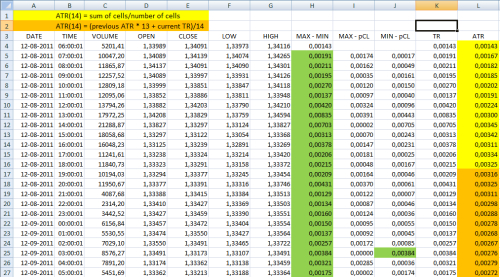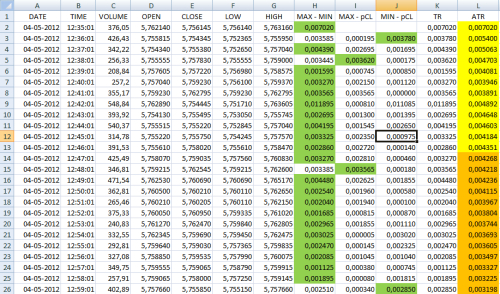Introduction

ATR indicator is the acronim for Average True Range. This indicator was developped by J. Welles Wilder. Wilder is a american mechanical engenieer who is the father of several other technical indicators: RSI (Relative Strenght Index), ADI (Average Directional Index) and Parabolic SAR (Stop and Reverse).  ATR is a volatility indicator. Volatility is a measure of the range of price action, but ignoring the price direction. Before was used the simple concept of "Range" that is the diference between the high/low values. Range values have some leaks, not considering gaps and limited price moves. Wilder created the concept of "TR - True Range" taking in consideration this leaks changing the way of how this values are calculated.

Calculating True Range

To calculate "True Range" we use the following formula:

Maximum value of this three calculations:
• Corrent High less current Low;
• Absolute value (without minus signal) of current High less previous Close ;
• Absolute value of current Low less previous close.
Or in a simple way:

TR = Max([High - Low], abs[High - previous Close], abs[Low - previous Close])

And as a image worth more than 1000 words:Image 1 - Some examples of TR calculation. TR value is represented by red lines with arrows. Simple Range value is represented by blue arrows.

Calculating Average True Range

ATR is calculated taking as base fo calculation previous ATR and actual TR. The value is calculated with the following formula taking in consideration a number N of periods:

Current ATR(N) = (Previous ATR x (N-1) + Current TR ) / N

The most used number of periods, and usually used by default, is 14 periods. As you can see, we talk about periods and not any time frame. This means that this indicator can be used with any time frame we want from ticks to years. Taking the default value as example and with last formula, we have:

ATR(14) = (Previous ATR x 13 + Current TR) / 14

If we look ate the formula, we have a problem when we need to start to calculate ATR because we need the previous value of ATR. This way, we need to calculate the first (N-1) ATR another way than the formula itself. To calculate the value of previous ATR needed for the formula, the first 13 ATR values are calculated the following way: first ATR value is the high - low of current bar, the next one is the sum of TR and the first value dividing by two, the next is the sum of two 2 TR (current and last) and the first value dividing by 3 and so on. We can see a example at next image.Image 2: Example or ATR calculation for a currency pair on 1 hour time frame. The yellow bckground values are calculated by the formula at top of table and the orange background with the ATR formula itself. The green background values are the TR values used for calculation.

The ATR concept is originaly developed for stocks and commodities markets. This markets only works during some hours of the day having a close period and on open at next day some prices have "gaps" (diference between close price last day and actual price at open - nº 1 in first image). WIth ATR we can handle correctly this gaps getting a more real "range". On Forex markets this situations occurs at weekend close/open and can occur also during trading period (on other markets too) with more frequency on currency pairs with high volatility and less volumes (we can see this in next image). Other thing that ATR handles is the "limited moves" - price action of current bar is "inside" the values of previous bar - as we can see in nº 4 at first image.Image 3: ATR calculations for other currency pair in one minute time frame. Here we can see some TR values don't are the values on MAX-MIN column but values of other columns.

ATR on charts

ATR represents the average "true range" what means in a simpler way the average move up or down in pips on each number of periods considered. As this value is absolute, usualy in charts is represented by a line where the reference or levels values represents the efective values of ATR. ATR values goes higher when we verify high volatility periods where the price action have greater moves. In other hand, the ATR values goes lower when the price action have lesser moves. We can see both situations in next image.Image 4: Reference absolute values on green circle. When the price action have lower moves we have lower ATR (leter A). When we have a price action with greater moves we obtain a greater ATR (leter B).

ATR usages

As any indicator, ATR don't is bullet proof, as is based on past data, but can give us some ideia about the volatility of the currency, stock or commodity we are observing. Greater ATR values for the time frame observed means that the price action have greater or faster moves but don't give us the direction of the move (up or down). We must consider, logically, that the higher the time frame in observation, the higher the ATR value. ATR can be useful in following situations:

• Mechanical strategies as a conditional trigger: by the strategies i have seen, usualy is used to trigger a entry if the values of ATR are getting bigger (in conjunction with other indicators). Also with great values than some defined limits can also avoid us from enter in a trade if we have higher volatility (equals higher ATR) in market instability periods. Other thing that can be done is adapting the strategy with the volatility taking diferent approachs for diferent interval values of ATR.
• Reversal moves approaching: If ATR is getting lesser values, that can mean we are approaching a reversal or trend weakness. Also can help us to identify "tops" or "bottoms" by the reasons mencioned before (we must refer that sometimes the inverse occurs if the market is experiencing a great volatility or instability period).
• Ranging markets: not a typo, but if we are in a ranging market usually we get low ATR values.
• Position sizing: taking in consideration ATR values for Stop Loss definition, and if we want to risk some defined % value of our account balance we can define the position size value for entry taking in consideration our money management.
• Defining Trailing Stop Loss: Some traders use ATR to help them to define the Trailing Stop Loss to avoid early exits on profitable trades. Higher ATR values means higher Trailing Stop Loss.
• Defining Stop Loss: Some traders use ATR to define Stop Loss taking in consideration ATR values and other factors. Higher ATR values usually means higher Stop Loss value.
• Defining Take Profit: taking in consideration other factors, ATR can help us to determine the Take Profit expectancy. In this case, sometimes are used higher time frames ATR values.
• To avoid bad entrys in a breakdown: in this case we wait the price moves some % percentage of ATR value at higher time frame below or above the S/R line to avoid to enter in correction moves. Works like a confirmation after the breakdown.
• ...
We can see that ATR is a useful indicator if used with other indicators or factors. By itself only give us the market volatility, but knowing this, that can be a hedge if we add it in our trading activity if we like to use indicators.  As always, none of the existing indicators or strategies are the "Holy Grail", but we can use them to help us or not depending the way we use them.

I end this article thanking all community the support and kind words that allowed me to be on the top 10 at this contest in last months. I want to extend this thanking to Dukascopy Team too. Thank you!

I hope you enjoy this article and find something useful in it. As always feel free to comment or send me a private message.